# 1 A) Which of the following pairs of solutions when mixed will form a buffer solution?...

1 A) Which of the following pairs of solutions when mixed will form a buffer solution? Group of answer choices H2SO4 and Ca(OH)2 NH3 and NH4Br NaNO3 and HNO3 KOH and KNO2

B)Phosphoric acid, H3PO4, is a triprotic acid with the following Ka values:

Ka1 = 7.5 x 10-3; Ka2 = 6.2 x 10-8; Ka3 = 3.6 x 10-13

In a solution of 0.15 M H3PO4, which species will be most abundant?

H3O+

H3PO4

HPO42-

H2PO4-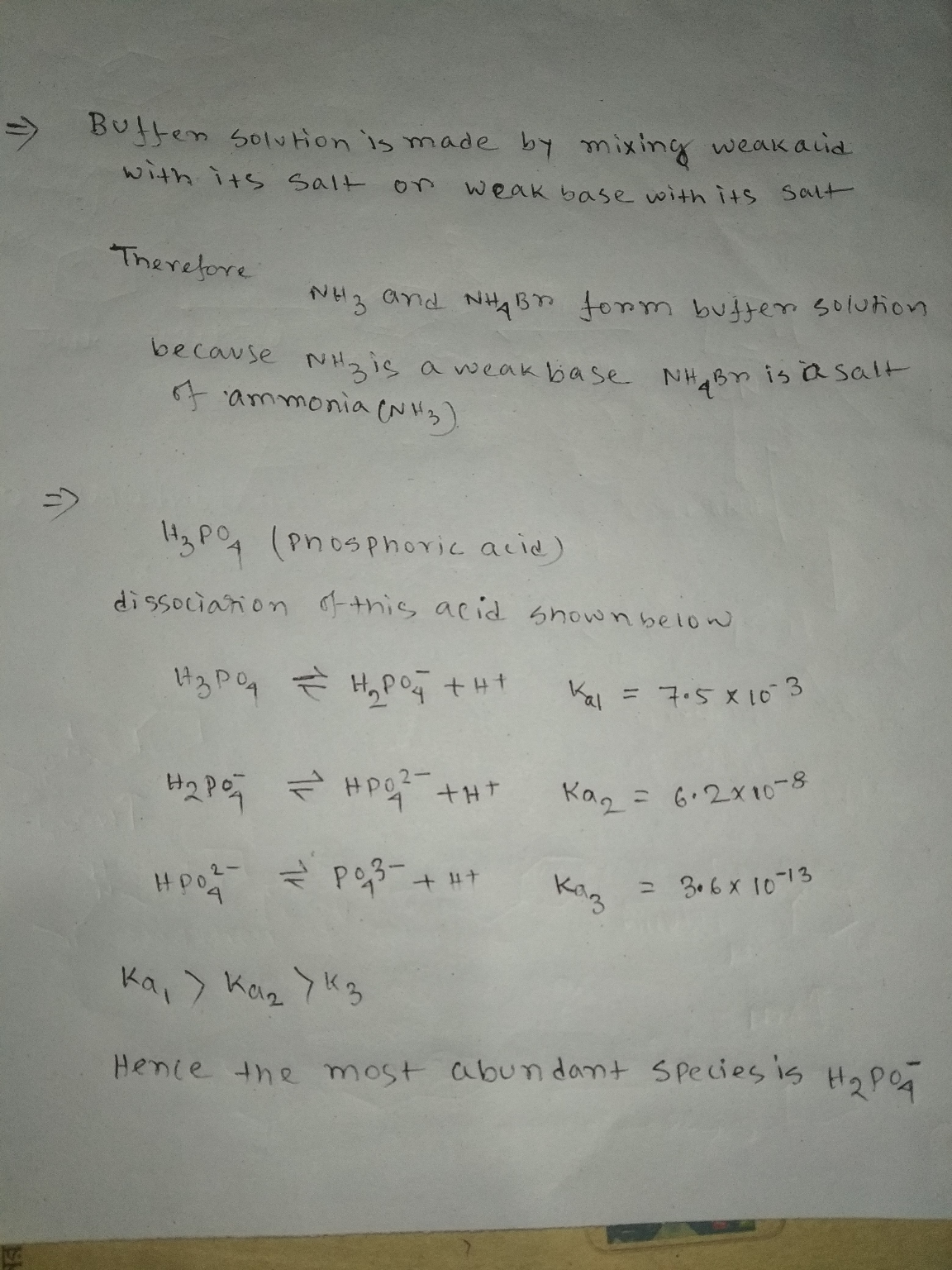#### Earn Coin

Coins can be redeemed for fabulous gifts.

Similar Homework Help Questions
• ### You are instructed to create 400. mL of a 0.40 M phosphate buffer with a pH...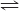You are instructed to create 400. mL of a 0.40 M phosphate buffer with a pH of 6.4. You have phosphoric acid and the sodium salts NaH2PO4, Na2HPO4, and Na3PO4 available. (Enter all numerical answers to three significant figures.) H3PO4(s) + H2O(l) H3O+(aq) + H2PO4−(aq)     Ka1 = 6.9 ✕ 10−3 H2PO4−(aq) + H2O(l) H3O+(aq) + HPO42−(aq)     Ka2 = 6.2 ✕ 10−8 HPO42−(aq) + H2O(l) H3O+(aq) + PO43−(aq) Ka3 = 4.8 ✕ 10−13 Which of the available chemicals will you use...

• ### You are instructed to create 200. mL of a 0.63 M phosphate buffer with a pH...

You are instructed to create 200. mL of a 0.63 M phosphate buffer with a pH of 6.0. You have phosphoric acid and the sodium salts NaH2PO4, Na2HPO4, and Na3PO4 available. (Enter all numerical answers to three significant figures.) H3PO4(s) + H2O(l) equilibrium reaction arrow H3O+(aq) + H2PO4−(aq) Ka1 = 6.9 ✕ 10−3 H2PO4−(aq) + H2O(l) equilibrium reaction arrow H3O+(aq) + HPO42−(aq) Ka2 = 6.2 ✕ 10−8 HPO42−(aq) + H2O(l) equilibrium reaction arrow H3O+(aq) + PO43−(aq) Ka3 = 4.8 ✕ ...

• ### Phosphoric acid, H3PO4, is a triprotic acid with the following acid dissociation constants: Ka1 = 7.5 × 10-3   Ka2 = 6.2...

Phosphoric acid, H3PO4, is a triprotic acid with the following acid dissociation constants: Ka1 = 7.5 × 10-3   Ka2 = 6.2 × 10-8   Ka3 = 4.2 × 10-13 Which of the following combinations would be best for preparing a pH 7 buffer? a. H3PO4 and NaH2PO4 b. H3PO4 and HCl c. Na2HPO4 and Na3PO4 d. NaH2PO4 and Na2HPO4 e. H3PO4 and Na3PO4

• ### You are instructed to create 400. mL of a 0.39 M phosphate buffer with a pH...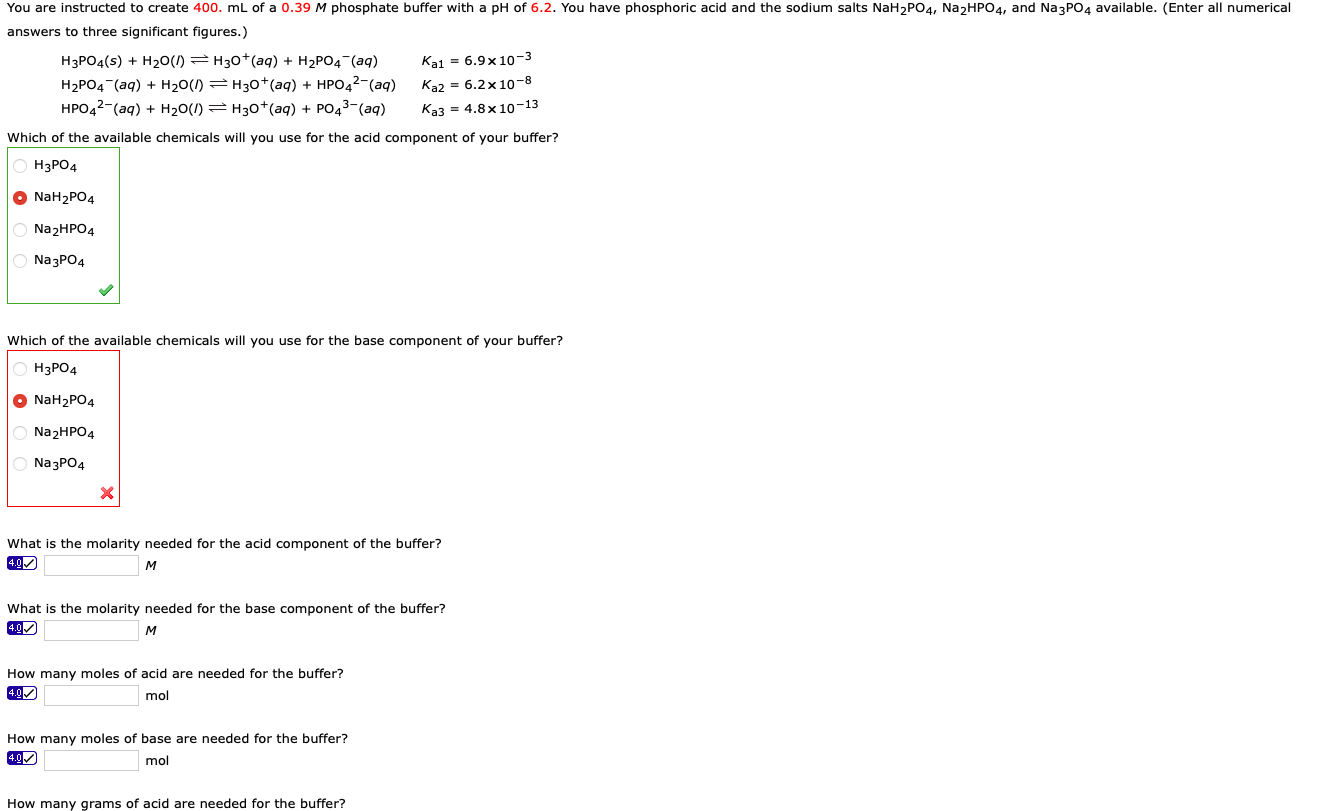You are instructed to create 400. mL of a 0.39 M phosphate buffer with a pH of 6.2. You have phosphoric acid and the sodium salts NaH2PO4, Na2HPO4, and Na3PO4 available. (Enter all numerical answers to three significant figures.) H3PO4(s) + H2O(l) = H30+ (aq) + H2P04 (aq) Kai = 6.9 x 10-3 H2PO4 (aq) + H20(1) =H30+ (aq) + HPO42-(aq) Ka2 = 6.2 x 10-8 HPO42-(aq) + H20(I) = H30+(aq) + PO43-(aq) Ka3 = 4.8 x 10-13 Which of...

• ### You are instructed to create 400. mL of a 0.39 M phosphate buffer with a pH...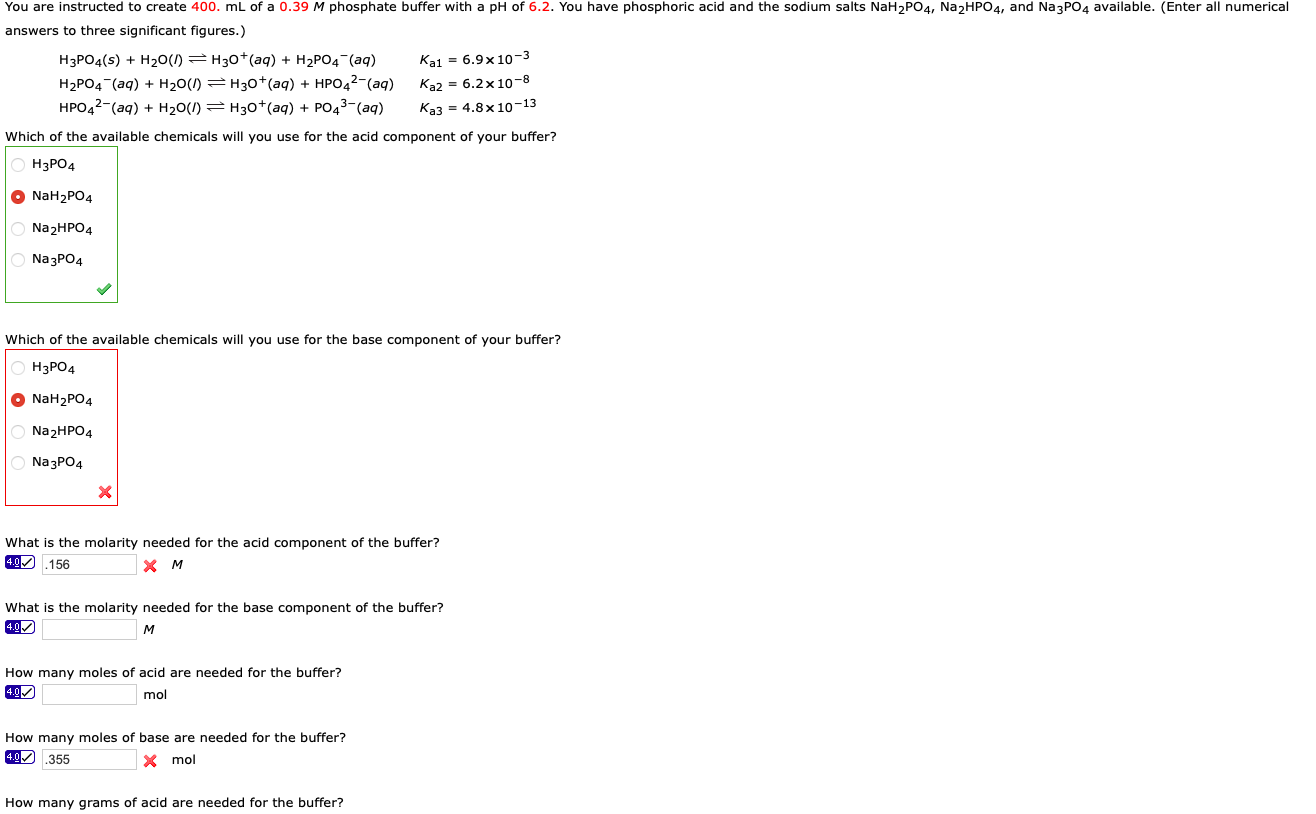You are instructed to create 400. mL of a 0.39 M phosphate buffer with a pH of 6.2. You have phosphoric acid and the sodium salts NaH2PO4, Na2HPO4, and Na3PO4 available. (Enter all numerical answers to three significant figures.) H3PO4(s) + H20(1) =H30+ (aq) + H2PO4 (aq) Kai = 6.9x10-3 H2PO4 (aq) + H20() = H30+(aq) + HPO42- (aq) Ka2 = 6.2x 10-8 HPO42-(aq) + H20(I) = H30+(aq) + PO43-(aq) Ka3 = 4.8 x 10-13 Which of the available chemicals...

• ### Worksheet 19 Titration of Polyprotic Acids Name: 1. Calculate the sulfite ion [SO3'] concentration in a...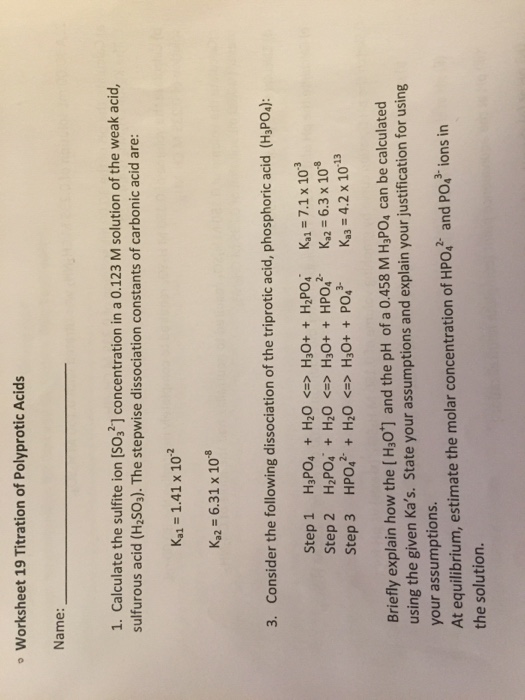Worksheet 19 Titration of Polyprotic Acids Name: 1. Calculate the sulfite ion [SO3'] concentration in a 0.123 M solution of the weak acid, sulfurous acid (H2SO3). The stepwise dissociation constants of carbonic acid are: Ka1 1.41 x 102 Ka2-6.31 x 108 3. Consider the following dissociation of the triprotic acid, phosphoric acid (HsPOs): Step 1 H3POa + H2O <=> H30+ + H2PO4, Step 2 HP0i + H2O <=> H30+ + HP042- Step 3 HPO42-+ H2O <-> H3O+ + PO43- Kal=7.1...

• ### A microbiologist is preparing a medium on which to culture E. coli bacteria. She buffers the medium at pH 7.00 to minimi...

A microbiologist is preparing a medium on which to culture E. coli bacteria. She buffers the medium at pH 7.00 to minimize the effect of acid-producing fermentation. What volumes of equimolar aqueous solutions of K2HPO4 and KH2PO4 must she combine to make 750.0 mL of the pH 7.00 buffer? Ka values for phosphoric acid: Ka1 = 7.2 ×10−3 Ka2 = 6.3 ×10−8 Ka3 = 4.2 × 10−13 Volume H2PO4− = ??? mL Volume HPO42− = ??? mL

• ### A buffer at pH 7.45 is prepared by mixing solutions of KH2PO4 and K2HPO4. Which of...

A buffer at pH 7.45 is prepared by mixing solutions of KH2PO4 and K2HPO4. Which of the following ratios of [base]/[acid] is required? For phosphoric acid, Ka1 = 7.5 × 10-3   Ka2 = 6.2 × 10-8   Ka3 = 4.2 × 10-13 [base]/[acid] = 1.75 [base]/[acid] = 1.24 [base]/[acid] = 0.57 [base]/[acid] = 1.27 [base]/[acid] = 0.79

• ### You are instructed to create 400. mL of a 0.39 M phosphate buffer with a pH...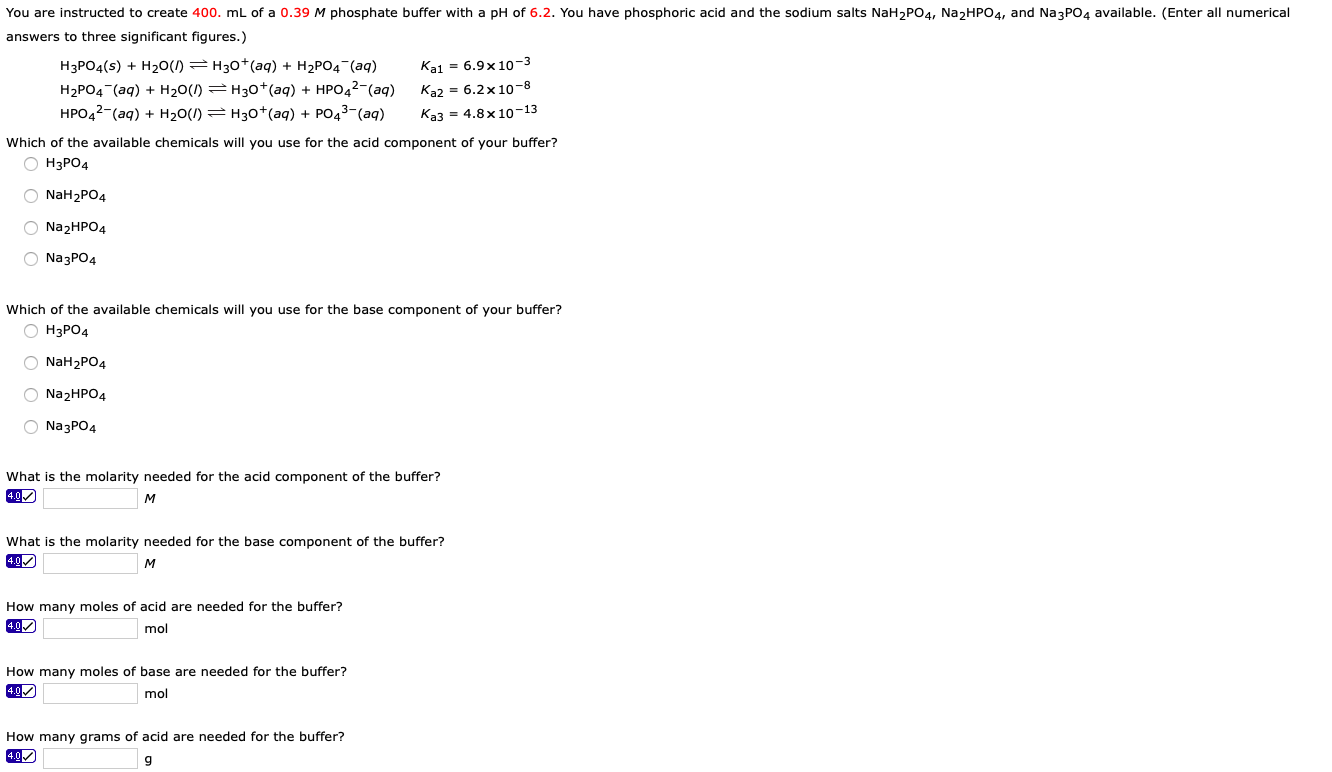You are instructed to create 400. mL of a 0.39 M phosphate buffer with a pH of 6.2. You have phosphoric acid and the sodium salts NaH2PO4, Na2HPO4, and Na3PO4 available. (Enter all numerical answers to three significant figures.) H3PO4(s) + H20(I) =H30+ (aq) + H2PO4 (aq) Kai = 6.9x10-3 H2PO4 (aq) + H20(1) =H30+ (aq) + HPO42-(aq) Ka2 = 6.2 x 10-8 HPO42-(aq) + H20(1) = H30+(aq) + PO43-(aq) Ka3 = 4.8x10-13 Which of the available chemicals will you...

• ### What is the concentration of phosphate ions in a 2.5 M solution of phosphoric acid?

What is the concentration of phosphate ions in a 2.5 M solution of phosphoric acid?(The pH of the solution is 0.87.)The acid-dissociation constants of phosphoric acid (H3PO4) at 25 degrees C are:Ka1 = 7.5 x 10-3Ka2 = 6.2 x 10-8Ka3 = 4.2 x 10-13I have tried finding this answer many times, but I am lost...Btw the answer is 1.9 x 10^-19